# Query Optimization¶

• Given a query written in logic, we would like to find out how to execute it as fast as possible, by taking into account:
• how the data is stored
• which query operations are involved and their various implementations
• Query optimization is one of the most complex tasks provided by the database, often one of the main reasons to use a database system for complex queries.
• Releaves the user from the burden to think about implementation and concentrate on logic (though you should really not write horrible queries)
• We will only give an overview of different steps, but the exact details are much more complex.

## Overview¶

• Query optimization is the process of taking a query written in SQL and converting to a query plan.

• A query plan is a query tree with:

• database tables are leaf nodes,
• each internal node is a query operator with an assignment of a specific implementation and memory allocation,
• and the root of the node is a correct implementation of the given query.
• Query optimization involves many different steps:

• Parse the query and rewrite if needed (for example eliminate nested correlated subexpressions if possible, simplify boolean expressions, etc.)

• Create a preliminary query tree

• Find access paths for selections (different indices that might apply)

Includes also potential sort operations if it can help the query for one or more operators

• Find different ways to join relations (which access paths to use and the join ordering) by enumerating all possible choices

Choose the lowest cost method by estimating the cost of each different option and eliminating options that cannot outpeform others

• Next, we will see each step.

## Query Parsing and Rewriting¶

• First, parsing checks to see queries are valid with respect to the table and attribute names, data types.

• Take view names and replace them with their definitions.

• Rewrite queries to simplify boolean expressions and resolve subexpressions that are always true or false.

SELECT A FROM R WHERE R.A > 5 AND R.A < 4;


returns nothing (the condition can never be true).

SELECT A FROM R WHERE R.B >= R.C OR R.C >= R.B;


equivalent to a simpler query:

SELECT A FROM R;

• More complex rewritings require semantic processing of the queries and schema.

Especially, try to rewrite nested queries without nesting if possible.

SELECT
r.a
, r.b
FROM
r
WHERE
r.a IN (SELECT s.a FROM s)


rewrite as:

SELECT
r.a
, r.b
FROM
r
,s
WHERE
r.a=s.a


but be careful about semantic equivalences. Are these two always equivalent?

• Suppose we are given:

SELECT
dept.name
FROM
dept
WHERE
dept.num-of-machines >=
(SELECT count(emp.*) FROM emp
WHERE dept.name = emp.deptname)


Is this equivalent to?

SELECT
dept.name
FROM
dept
, emp
WHERE
dept.name = emp.dept-name
GROUP BY
dept.name, dept.num-of-machines
HAVING
dept.num-of-machines >= count(emp.*)


## Query Trees¶

• An SQL query is translated to an equivalent query tree.
• A query treee has
• Tables in the query as leaf nodes
• Relational algebra operators as nodes
• The root of the tree returns the correct results for the given query.
• A single query can have many equivalent query trees due to the properties of the relational algebra operators.
• The query optimizer will consider all potential query trees when deciding how to optimize the query.

## Query Rewriting Rules (Algebraic Equivalences)¶

• Suppose we are given R(A,B,C) and S(D,E,F).

### Selections¶

• Selections can be pushed through joins and Cartesian products.Note that the selection over R also results in a selection over S.

• The reverse is also true: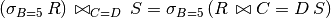First, push all selections high up the tree and push them all the way down.

• Note that selections open up different access paths to the relation (i.e. indices) and can significantly reduce the size of joins.

• There are cases in which applying some selections early may not be desirable. We will use cost to guide when to do this.

• Selections can be joined with Cartesian products for a join condition: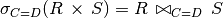### Projections¶

• Projections can be pushed through joins, Cartesian products to reduce the size of the output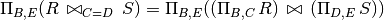Simply remove attributes not needed in higher level nodes at each step.

Even though the number of tuples do not change (bag projection), the length of each tuple is smaller and more tuples can fit in a single memory block.

### Joins¶

• Joins are associative and commutative (they can be shuffled).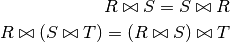We will see join ordering is a crucial part of query optimization shortly.

## Cost-based Query Optimization¶

• Given alternate query trees, find the lowest cost one.

• We do not really need to generate all possible query trees, but find:

• All potential access paths for selections (indices and table scans)
• Join orderings that combine these access paths with specific join implementations
• Estimate the cost of a partial query plan and eliminate other plans that can never be cheaper.
• To be able to estimate the cost of query plans, we need to know:

• Cardinality estimation: How many tuples we expect as the output of joins and selections

• Space estimation: The size of the tuples on disk to estimate how many disk pages are needed to store them.

Space estimation is easy due to the knowledge of schema. We will disregard this for now.

## Database Statistics¶

• Compute database statistics regarding each table. This can be accomplished using the Analyze command in SQL.

• Simplest statistics:

• TUPLES(R): the number of tuples in R
• PAGES(R): the number of pages R spans
• N_DISTINCT(R.A): the number of distinct values stored for R.A
• MINVAL(R.A)/MAXVAL(R.A): min/max values stored for R.A
• You can query these statistics also using Postgresql system tables.

select
relname
, relpages
, reltuples
from
pg_class pc
, pg_user pu
where
pu.usename=user
and pc.relowner = pu.usesysid ;

select
attname
, inherited
, n_distinct
, histogram_bounds
, array_to_string(most_common_vals, E'\n') as most_common_vals
from
pg_stats
where
tablename = 'movieroles';


## Cardinality estimation¶

• For any condition, we can estimate the size of the output using the given statistics

• Statistics must be updated periodically to be useful
• The more statistics you keep, better the size estimation. However, cost of updating them goes up.
• sel(Cond), selectivity of c is the percentage of tuples that will satisfy a condition Cond.

The more selective conditions have lower selectivity values.

• exp(X), the expected number of tuples in a relation X depends on the operations used to define X.

• Example: selection estimation:exp(X) = sel(Cond) * TUPLES(R)

• Example: join estimation:exp(Y) = sel(JCond) * (TUPLES(R)*TUPLES(S))


find the selectivity of the join condition, and multiply it with the number of expected tuples in the Cartesian product.

## Size estimation for equality¶

• Only simple rules can be applied for simpler statistics.

• Given any equality condition on a constant, assume each constant is equally likely (uniform distribution):

sel(R.A=c) = 1/N_DISTINCT(R.A)

• For example:

sel(student.year='freshman') = 1/4


Assuming 4 different years and equal number of students in each year.

sel(student.major) = 1/N_DISTINCT(student.major)


Assuming each major is equally likely: same number of students in each major.

• Note that uniform distribution is generally not true. For example, there may be a few large majors in the university and a number of small ones.

• So, we will underestimate the counts for large majors and overestimate for small majors.
• This is the best we can do with a single statistic. If not useful, we need better statistics.

## Size estimation for joins¶

• Simple join conditions join across attributes from two tables.

sel(R.A = S.B)


You can look at this as one of two conditions:

1. sel(R.A = c1) = 1/N_DISTINCT(R.A)
2. sel(c2 = S.B) = 1/N_DISTINCT(S.B)


Of these two, we want to choose the most selective one as the dominant selection condition.

sel(R.A = S.B) = 1/( MAX{ N_DISTINCT(R.A), N_DISTINCT(S.B) } )


## Size estimation for ranges¶

• You can approach ranges as a collection of individual values, but this would be wrong when the values are over a large range:

select( 3.0 <= students.gpa <= 3.5 )
select( c1 <= employee.salary <= c2 )


For GPA, there may be many distinct values as this is a float. So, you cannot treat it like a collection of distinct values.

For salary, there may be very few distinct values of salary, but the range requested may be large, larger than even the number of distinct values.

• Instead, we can approach ranges as a slice over a continuous range:

sel( c1 <= R.A <= c2 ) = (c2-c1)/(maxval(R.A)-minval(R.A))


This could be very wrong if the range is very large but most values are clustered around a narrow range, i.e. large skew.

For example: average wealth of top 400 richest Americans have is $5.8B (10^9), while median (typical) wealth of an American is$44K (10^3).

• Alternatively, you can use a simple formula to assume that most range searches are not very selective:

sel( c1 <= R.A <= c2 ) = 1/3


This is a simple rule that will stand in for unselective ranges. It might be better to use this instead of an incorrect estimate.

• If the ranges are crucial to your query, and some ranges are selective while some are not, you need to use a better statistic.

• Note that string match operations:

  name like 'abc%'
name like '%abc%'

are also range searches. Cardinality estimation is even more
complex for these and use of the simple formula is more appropriate
in these cases.


## Histograms¶

• Histograms divide values an attribute take into ranges and count for each range, how many tuples are in that range

• Equal width histograms find the ranges such that there are equal number of tuples in each range:

select
avg_width
, histogram_bounds
from
pg_stats
where
tablename = 'imdbratings'
and attname = 'rating';


will give us:

{1.6,2.3,2.9,3.5,3.7,3.9,4,4.2,4.3,4.4,4.5,4.6,
4.6,4.7,4.8,4.8,4.9,4.9,4.9,5,5,5.1,5.1,5.1,5.2,
5.2,5.2,5.2,5.3,5.3,5.3,8.5,8.5,8.5,8.5,8.7,8.7,
8.7,8.8,8.8,8.8,8.9,9,9.1,9.2,9.5,10}

• To estimate the selectivity for a range query:

sel( 8.0 <= imdbratings.rating <= 9.0 )


Find the histogram ranges that overlap with the given range, find overlap for each range and divide by the total number of histogram ranges.

For example, it would be about 1/4 of tuples in this case.

• Note that Postgresql will store the most frequent values and their frequency separately of the histogram (see 5.3-8.5 range for example) and use them in estimates for such values:

select
most_common_vals
, most_common_freqs
from
pg_stats
where
tablename = 'imdbratings'
and attname = 'rating';


## Selectivity of Boolean Conditions¶

• We will use simple probabilistic interpretation of selectivities:

sel(Cond): probability that a tuple will be put in the output

• Using this, we can come up with the following rules:

sel(not Cond) = 1 - sel(Cond)

sel(Cond1 and Cond2) = sel(Cond1) * sel(Cond2)

sel(Cond1 or Cond2) = 1 - ((1 - sel(Cond1)) * (1 - sel(Cond2)))

• First, we see that negation is not generally selective.

• Second, AND conditions are treated as if two conditions are independent of each other.

This is often not true:

sel(voting_district=c1 and vote=c2)


Certain districts will vote predominantly for one party over the other. Counting votes like this will not work.

• Third, OR conditions are treated as

Cond1 OR Cond2 = NOT ((NOT Cond1) AND (NOT Cond2))


However this double counts tuples that satisfy both Cond1 and Cond2.

## Cardinality Estimation Summary¶

• Cardinality estimation is rough and can make many mistakes.

• Often, we do not care for the errors:

• The big errors may apply to rarely queried values, then they have little impact.

• The actual numbers are not important. Query optimization is about comparing magnitude of different plans.

As long as the estimates reflect the ordering with respect to cost, then they are still useful.

• In case we care about the errors, use more sophisticated statistics but keep them up to date for most effect.

## Cardinality Estimation Examples¶

• Let’s estimate the size of the following queries:

Q1: SELECT * FROM R WHERE R.A = 5 and R.B < 10

sel(R.A=5) = 1/N_DISTINCT(R.A)
sel(R.B<10) = 1/3  (use simple heuristics)

sel(Q1) = 1/(3*N_DISTINCT(R.A))

exp(Q1) = TUPLES(R)/(3*N_DISTINCT(R.A))

Q2: SELECT * FROM R WHERE R.C=5 AND R.D=10 AND R.E NOT LIKE 'e%';

exp(Q2) = 2/ (N_DISTINCT(R.C)*N_DISTINCT(R.D)*3)


Note that the selectivity of NOT LIKE is estimated to be 1-1/3=2/3.

• Joins:

Q3: SELECT * FROM R,S WHERE R.A=S.B

exp(Q3) = (TUPLES(R)*TUPLES(S)) / max(N_DISTINCT(R.A), N_DISTINCT(S.B))

Q4: SELECT * FROM S,T WHERE S.X<T.Y AND T.Z=5

exp(Q4) = (TUPLES(S)*TUPLES(T)) / (3*N_DISTINCT(T.Z))


Note that the join selectivity is assumed to be 1/3 due to the inequality.

## Index Selectivity¶

• Index selectivity is an important measure of usefulness of indices

• The index selectivity for a selection query is the portion of the query that is answered by the index

• Note that overall the cost of the index use must be used in understanding how useful an index is

• Example:

SELECT * FROM R WHERE R.A=5 AND R.B=5 AND R.C>3

Index I1 on R(A,B) will be used for R.A=5 AND R.B=5 potentially
very selective conditions (depending on the number of distinct
values

Index I2 on R(D,A,B) has the same selectivity as I1, but we will
need to scan the whole leaf level to find these tuples

Index I3 on R(C,B) is potentially less selective as the only
conditions R.B=5 AND R.C>3 can be applied and a great deal of the
index must be searched.

You can also combine two indices: I1 and I3: Look up tuples that
satisfy conditions supported by these indices and take their
intersection in memory, before reading the found tuples from data
pages.

• Indices can be ordered in general for their selectivity and usefulness

• A clustered index on one or more attributes, for example, R(A,B) is useful for searching equality or range on A and B because tuples with the same A,B values are clustered.

This is potentially the most useful index. However, you can only create one cluster for a table.

• An unclustered/secondary index on attributes often searched for equality on selective attributes is very useful. A special case of this is the primary key. An index is usually automatically created for the primary key by most databases.

• An unclustered index to be used on less selective conditions can be useful if the index contains all the attributes needed for a query. In this case, query can be answered using the index only.

For this to work, the size of the index on leaf nodes should be considerably smaller than the table.

SELECT C FROM S WHERE B<5

An index on S(B,C) can be very useful for this query even if
not very selective.


## Cost-Based Query Optimization¶

• Generate and estimate the cost of different access paths to base relations (with or without using different indices)

• Consider sorting as an option, even though it is an upfront cost, it might help other operations up the query tree.

• Incorporate projections when possible to reduce size of the tuples and other methods to reduce size of relations that can be done on the fly:

On the fly operations do not require additional cost. They apply to tuples as they are produced in memory.

• Evaluate all possible ways to combine these relations by considering join orderings using dynamic programming:

Consider all two way join orderings to construct a partial query tree
Estimate the cost so far

While there are joins to add:
Consider adding a new join to a partial query tree
and estimate cost

Remove all query trees with fewer joins that are costlier
(because adding a join will only increase the cost)
with the exception of trees involving sorts (their benefit
may show later)


## Join orderings¶

• Considering all possible join orderings may be too costly.

• For a three relation join, there are many options:

(R join S) join T        R join (S join T)
(S join R) join T        S join (R join T)
(T join S) join R        T join (S join R)
(S join T) join R        S join (T join R)
(R join T) join S        R join (T join S)
(T join R) join S        T join (R join S)

• We need to decide on the shape of join tree, the ordering of the joins and then decide inner/outer relations for each join.

• Main join tree types: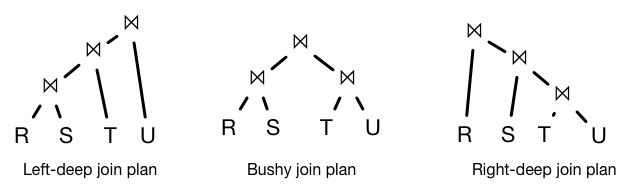• Left-deep joins are particularly useful because the output of one operation can be pipelined into the next join operation

• Bushy joins can be very useful when relations are stored in different disks or machines, allowing the database to use parallelism in computing the query.

In cases where relations are remote, other operations are introduced such as semi-joins, that filter for key values that will participate in joins before shipping data across a network.

• In this class, we will assume only left-deep join trees with pipelined operations.

The relation ordering (inner/outer) is determined by the underlying join operation. Often it is beneficial to put smaller relation as the outer relation, reducing the number of passes over the inner relation.

## Join ordering example¶

• Suppose we have the following statistics for the following query:

SELECT R.E,S.F,T.G FROM R,S,T WHERE R.A=S.B AND S.C=T.D

RELATION TUPLES PAGES
R 10,000 500
S 200,000 1,000
T 50,000 2,000
ATTR N_DISTINCT
R.A 10,000
S.B 9,000
S.C 500
T.D 800
• Let’s disregard the size reduction that can be obtained through projections. Assume that after a join, we can fit about 50 tuples per page.

We will only consider block nested loop joins. Assume each operation has M=101 pages.

exp(R join S) = 10,000*200,000/10,000 = 200,000 (tuples fits in 2,000 pages)

Block-nested loop join: 500 + 5*1,000 = 5,500 pages

exp(S join T) = 200,000*50,000/800= 12,500,000 tuples (fits in 250,000 pages)

Block-nested loop join: 1,000 + 10*2,000 = 21,000 pages

exp(R join T) = 10,000*50,000 = 500,000,000 tuples (fits in 10,000,000 pagees)

Note that this is a Cartesian product as there are no join conditions
between R and T.

Block-nested loop join: 500 + 5*2,000 = 10,500 pages

• Now, we will see how to add a third join. Let’s start with the cheapest operation and go forward.

exp( (R join S) join T ) = 200,000*50,000/800= 100000000/8 =
12,500,000 tuples (note: this is the same for all the different
plans, so we will not compute this for the rest.)

Read R join S into 100 pages, and read T into the remaining.

T is read: 2,000/100 = 20 times (size of R join S fits in 2,000 pages)

No additional cost to read R join S, the tuples are in memory as
it is being pipelined from the operator below:

Total cost = (Cost of R join S) + (Cost of reading T 20 times for the last join)
Total cost = 5,500 + 20*2,000 = 45,500 pages

• Let’s see the same for other joins:

Cost of (S join T) join R = 21000 + 2500*500 = 1,271,000

Cost of (R join T) join S = 10500 + 100000*1000 = 100,010,500

• The best plan is: (R join S) join T